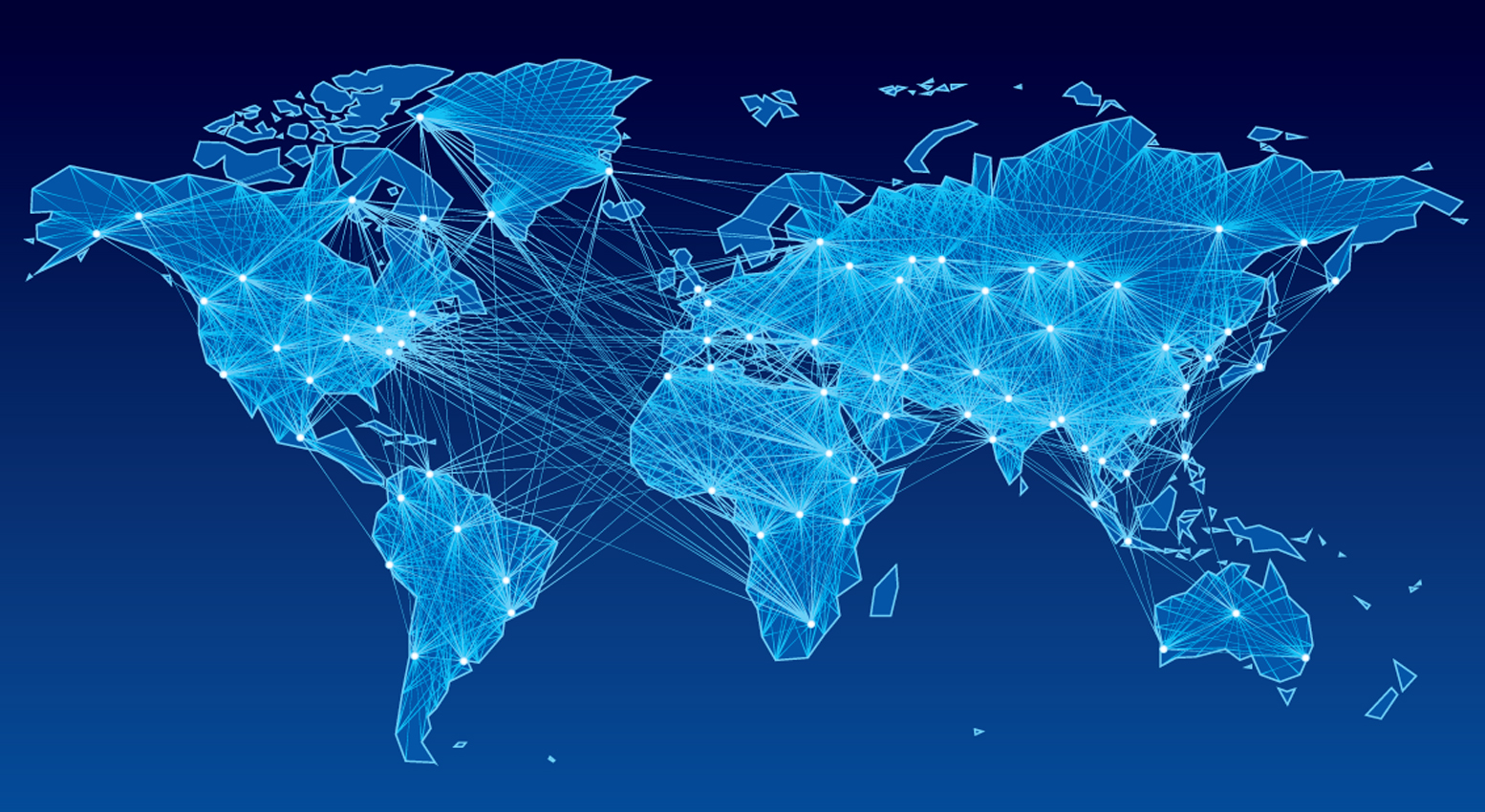# Friends at infinity

Probability Level 4Take a simple model of a social network where friendships form at random between individuals. Each person forms some number of friendships with other people, $k_i$. The average number of friendships that any given person makes is then $\frac{1}{N}\sum\limits_ik_i = \langle k \rangle$.

We call a friendship island (FI) a group of people such that everyone in the FI can reach anyone else in the FI by passing a note through mutual friends. If two people cannot send notes through a series of mutual friends, they must be in different FI.

At some value of $\langle k \rangle$, $\langle k\rangle_c$, the expected size of the largest FI becomes $\infty$. What is the value of $\langle k \rangle_c$?

Notes and assumptions

• There are infinitely many people in the population.
×